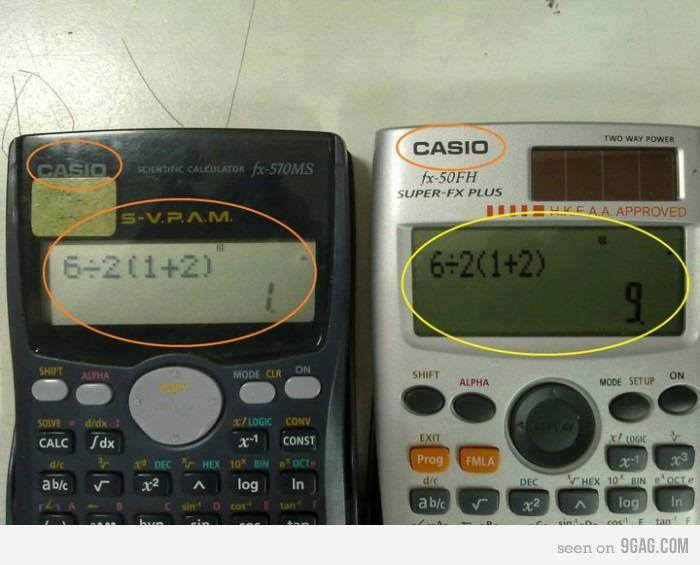# When calculators get it very wrong! Can you explain this?When calculators get it terribly wrong! Can you explain this?

This is definitely an example of when calculators get it very wrong and would make a great starter for discussion and debate in any Maths lesson. It appears that one of the calculators has not been taught the order of operations, can you tell which one? : )Can you explain why this is the case? The original tweet caused a twitter storm and generated some interesting discussions. Comments are free, please leave one below.###### 6 comments on “When calculators get it very wrong! Can you explain this?”
1.Andrew says:

EMP

2.Miss Davies says:

One worked left to right, the other has done the division last.

3.Angela Little says:

The 2nd is using BODMAS (or Order of Operations) which should be used. The first is just going from left to right.

4.Frank Boulton says:

The second uses the BODMAS rule, which is what I was taught at school. This is the correct way. The first seems to work from left to right. However, I wonder if the difficulty might lie in the fact that the expression has an implied but unwritten multiplication sign before the left parenthesis. I wonder what would happen with the first calculator, if we explicitly entered that multiplication sign.

5.Heidi says:

It’s not simply because the first calculator has read left to right. It’s because the programming in the first for BIDMAS (UK version of BODMAS) is wrong. The first calculator works out 1 + 2 first, giving 3, so the brackets have the correct priority. Then it multiplies the 3 in the brackets by 2, giving 6. So it is not reading left to right but is prioritising multiplication over division in the second level, as it is following BIDMAS literally, i.e. B, I, D, M, A, S, instead of giving equal priority to division and multiplication (DM). This gives an answer of 6, which is then divided by 6, giving 1 as the answer.

If you read left to right, it would be 6 divided by 2 (equal 3) times 1 (equal 3) plus 2 (equal 5).

6.Heidi says:

It’s not simply because the first calculator has read left to right. It’s because the programming in the first for BIDMAS (UK version of BODMAS) is wrong. The first calculator works out 1 + 2 first, giving 3, so the brackets have the correct priority. Then it multiplies the 3 in the brackets by 2, giving 6. So it is not reading left to right but is prioritising multiplication over division in the second level, as it isn’t following BIDMAS literally, i.e. B, I, D, M, A, S giving equal priority to division and multiplication (DM). This gives an answer of 6, which is then divided by 6, giving 1 as the answer.

If you read left to right, it would be 6 divided by 2 (equal 3) times 1 (equal 3) plus 2 (equal 5).